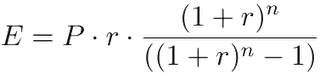# EMI Calculator for Home Loan, Car Loan & Personal Loan in India

The results of this loan payment calculator are for comparison purposes only. They will be a close approximation of actual loan repayments if available at the terms entered, from a financial institution. This is being provided for you to plan your next loan application. To use, enter values for the Loan Amount, Number of Months for Loan, and the Interest Rate (e.g. 7.25), and click the Calculate button. Clicking the Reset button will clear entered values.

## EMI Calculator for Home Loan, Car Loan & Personal Loan in India

Description Data Entry
Loan Amount
Loan Length in Months
Interest Rate
Monthly PaymentCalculated
Enter only numeric values (no commas), using decimal points where needed.
Non-numeric values will cause errors.

## What is EMI?

Equated Monthly Installment – EMI for short – is the amount payable every month to the bank or any other financial institution until the loan amount is fully paid off. It consists of the interest on loan as well as part of the principal amount to be repaid. The sum of principal amount and interest is divided by the tenure, i.e., number of months, in which the loan has to be repaid. This amount has to be paid monthly. The interest component of the EMI would be larger during the initial months and gradually reduce with each payment. The exact percentage allocated towards payment of the principal depends on the interest rate. Even though your monthly EMI payment won’t change, the proportion of principal and interest components will change with time. With each successive payment, you’ll pay more towards the principal and less in interest.
Here’s the formula to calculate EMI:where
E is EMI
P is Principal Loan Amount
r is rate of interest calculated on monthly basis. (i.e., r = Rate of Annual interest/12/100. If rate of interest is 10.5% per annum, then r = 10.5/12/100=0.00875)
n is loan term / tenure / duration in number of months
For example, if you borrow ₹10,00,000 from the bank at 10.5% annual interest for a period of 10 years (i.e., 120 months), then EMI = ₹10,00,000 * 0.00875 * (1 + 0.00875)120 / ((1 + 0.00875)120 – 1) = ₹13,493. i.e., you will have to pay ₹13,493 for 120 months to repay the entire loan amount. The total amount payable will be ₹13,493 * 120 = ₹16,19,220 that includes ₹6,19,220 as interest toward the loan.